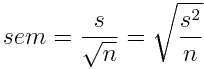The standard error of the mean is a measure of how much random sampling error is likely to be in a sample mean. That is, how far is the sample mean likely to be from the true population mean due to random sampling error?All statistics have a standard error, but the standard error of the mean is the one most often calculated and virtually the only one ever reported as a descriptive statistic. To be technically correct, the calculation above is of the estimated standard error of the mean, but the distinction is rarely made (or necessary) outside of mathematical statistics. Return To Main Page